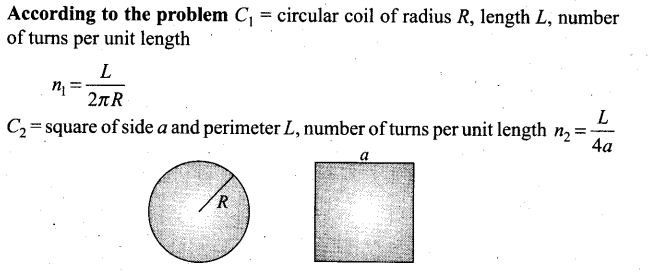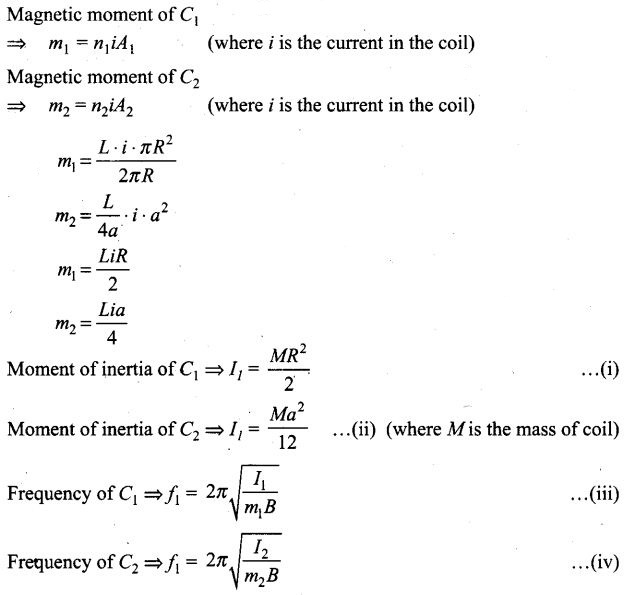# Magnetism and Matter NCERT Exemplar Problems Solutions Physics

0
2201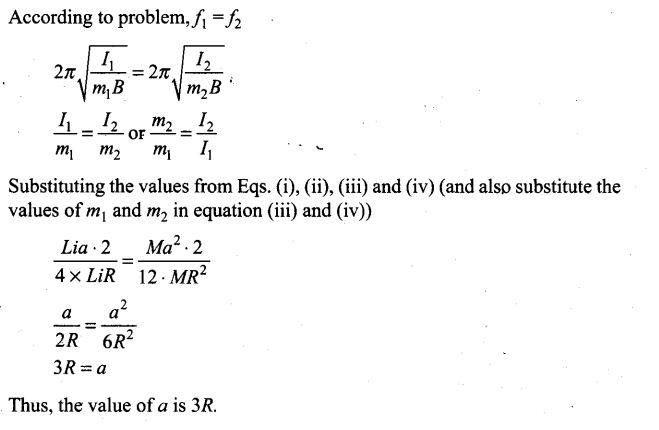## NCERT Exemplar Problems Class 12 Physics Chapter 5 Magnetism and Matter

Multiple Choice Questions (MCQs)
Question 1. A toroid of n turns, mean radius R and cross-sectional radius a carries current I. It is placed on a horizontal table taken as xy-plane. Its magnetic moment m
(a) is non-zero and points in the z-direction by symmetry
(b) points along the axis of the toroid (m = mФ)
(c) is zero, otherwise there would be a field failing as 1/r3 at large distances outside the toroid
Solution: (c)
Key concept: Toroid’. A toroid can be considered as a ring shaped closed solenoid. Hence it is like an endless cylindrical solenoid.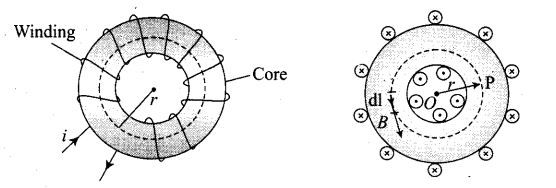The magnetic field is only confined inside the body of a toroid in the form of concentric magnetic lines of force. For any point inside the empty space surrounded by toroid and outside the toroid, the magnetic field B is zero because the net current enclosed in these spaces is zero. Thus, the magnetic moment of toroid is zero.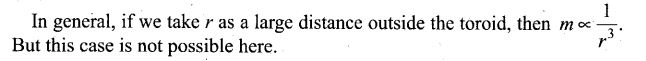Question 2. The magnetic field of the earth’ can be modelled by that of a point dipole placed at the centre of the earth. The dipole axis makes an angle of 11.3° with the axis of the earth. At Mumbai, the declination is nearly zero. Then
(a) the declination varies between 11,3°W to 11.3°E
(b) the least declination is 0°
(c) the plane defined by dipole axis and the earth axis passes through Greenwich
(d) declination averaged over the earth must be always negative
Solution: (a) The magnetic field lines of the earth resemble that of a hypothetical magnetic dipole located at the centre of the earth.
The axis of the dipole does not coincide with the axis of rotation of the earth and it is tilted at some angle (angle of declination). Here in this situation the angle of declination is approximately 11.3° with respect to the later. Here two possibilities arises as shown in the figure below.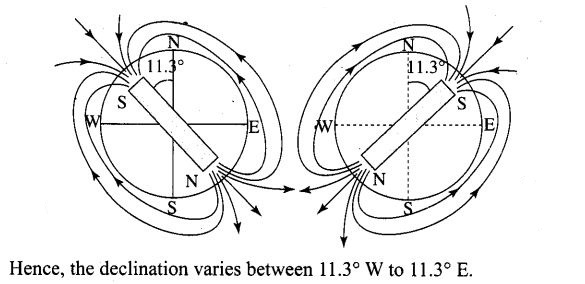Question 3. In a permanent magnet at room temperature,
(a) magnetic moment of each molecule is zero
(b) the individual molecules have non-zero magnetic moment which are all perfectly aligned
(c) domains are partially aligned
(d) domains are all perfectly aligned
Solution: (d)
Key concept: At room temperature permanent magnet behaves as a ferromagnetic substance for a long period of time.
At room temperature, the permanent magnet retains ferromagnetic property for a long period of time.
The individual atoms in a ferromagnetic material possess a dipole moment as in a paramagnetic material.
However, they interact with one another in such a way that they spontaneously align themselves in a common direction over a macroscopic volume called domain. Thus, we can say that in a permanent magnet at room temperature, domains are all perfectly aligned.

Question 4. Consider the two idealised systems (i) a parallel plate capacitor with large plates and small separation and (ii) a long solenoid of length L>>R, radius of cross-section. In (i) E is ideally treated as a constant between plates and zero outside. In (ii) magnetic field is constant inside the solenoid and zero outside. These idealised assumptions, however, contradict fundamental laws as below: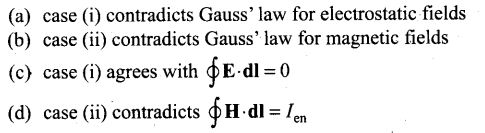Solution: (b)
Key concept: The electrostatic field lines, do not form a continuous closed path (this follows from the conservative nature of electric field) while the magnetic field lines form the closed paths.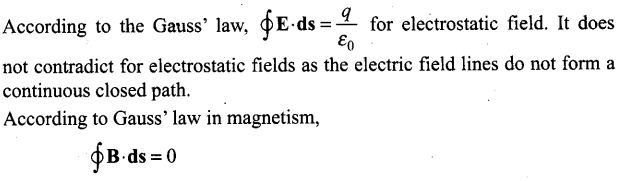Which implies that number of magnetic field lines entering the Gaussian surface is equal to the number of magnetic field lines leaving it. Therefore case (ii) is not possible.

Question 5. A paramagnetic sample shows a net magnetisation of 8 Am-1 when placed in an external magnetic field of 0.6 T at a temperature of 4 K. When the same sample is placed in an external magnetic field of 0.2 T at a temperature of 16 K, the magnetisation will be
(a) 32/3 Am-1 (b) 2/3 Am-1
(c) 6 Am-1 (d) 2.4 Am-1
Solution: (b)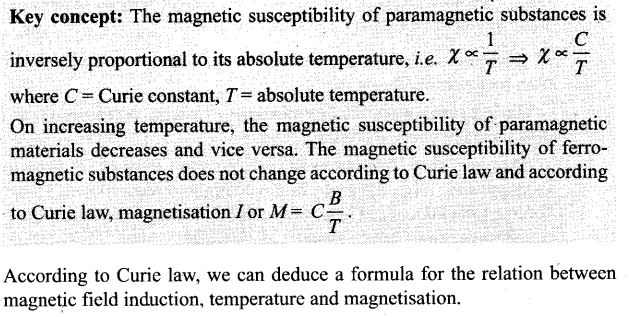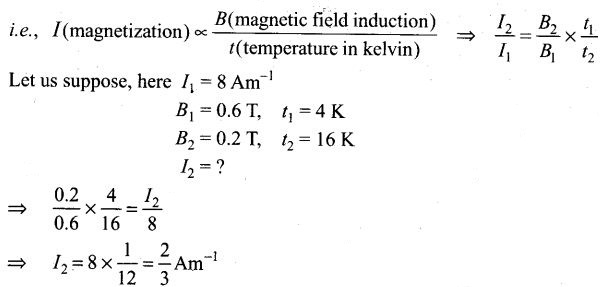One or More Than One Correct Answer Type
Question 6. S is the surface of a lump of magnetic material.
(a) Lines of B are necessarily continuous across S
(b) Some lines of B must be discontinuous across S
(c) Lines of H are necessarily continuous across S
(d) Lines of H cannot all be continuous across S
Solution: (a, d)
Key concept: Here we are introducing properties of magnetic field lines (B), for any magnet, it forms continuous closed loops. This is unlike the electric dipole where these field lines begin from a positive charge and end on the negative charge or escape to infinity.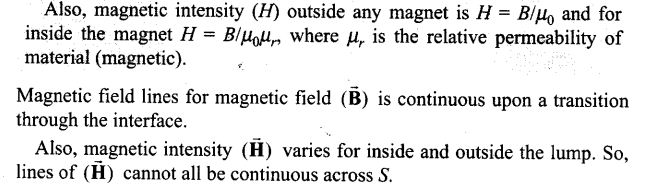Question 7. The primary origin(s) of magnetism lies in
(a) atomic currents (b) Pauli exclusion principle
(c) polar nature of molecules (d) intrinsic spin of electron
Solution: (a, d) The primary origin of magnetism lies in the fact that the electrons are revolving and spinning about the nucleus of an atom, and we know that an moving charge carries current along with it. We meant this current here as atomic current and which is responsible to produce an orbital magnetic moment. This atomic current gives rise to magnetism. The revolving and spinning about nucleus of an atom is called intrinsic spin of electron, which gives rise to spin magnetic moment. So, total magnetic moment is the sum of orbital magnetic moment and spin magnetic moment.

Question 8. A long solenoid has 1000 turns per metre and carries a current of 1 A. It has a soft iron core of μr = 1000. The core is heated beyond the Curie temperature, Tc.
(a) The H field in the solenoid is (nearly) unchanged but the B field decreases drastically
(b) The H and B fields in the solenoid are nearly unchanged
(c) The magnetisation in the core reverses direction
(d) The magnetisation in the core diminishes by a factor of about 108
Solution: (a, d)
Key concept: The magnetic field intensity H = nl, where n = number of turns per metre of a solenoid and I = current and B =μ0μrI.
Also, at normal temperature, a solenoid behaves as a ferromagnetic substance and at the temperature beyond the Curie temperature, it behaves as a paramagnetic substance.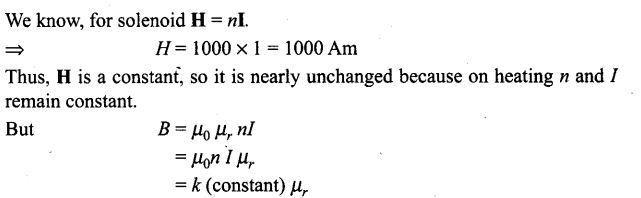but there is a large decrease in the susceptibility of the core on heating it beyond critical temperature, hence magnetic field will decrease drastically. Now, for magnetisation in the core, when temperature of the iron core of a solenoid is raised beyond Curie temperature, then it behaves as a paramagnetic material, where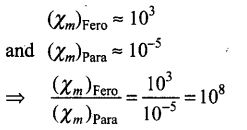Question 9. Essential difference between electrostatic shielding by a conducting shell and magnetostatic shielding is due to
(a) electrostatic field lines can end on charges and conductors have free charges
(b) lines of B can also end but conductors cannot end them
(c) lines of B cannot end on any material and perfect shielding is not possible
(d) shells of high permeability materials can be used to divert lines of B from the interior region
Solution: (a, c, d)
Electrostatic shielding is the phenomenon to block the effects of an electric field. The conducting shell can block the effects of an external field on its internal content or the effect of an internal field on the outside environment. For protecting a sensitive equipment from the external magnetic field it should be placed inside an iron cane (magnetic shielding). Magnetostatic shielding is done by using an enclosure made of a high permeability magnetic material to prevent a static magnetic field outside the enclosure from reaching objects inside it or to confine a magnetic field within the enclosure.

Question 10. Let the magnetic field on the earth be modelled by that of a point magnetic dipole at the centre of the earth. The angle of dip at a point on the geographical equator (a) is always zero (b) can be zero at specific points (c) can be positive or negative (d) is bounded
Solution: (b,c,d)
Key concept: Angle of inclination or dip is the angle between the direction of intensity of total magnetic field of the earth and a horizontal line in the magnetic meridian.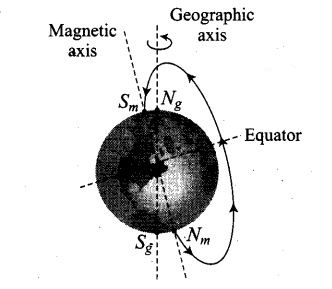If the total magnetic field of the earth is modelled by a point magnetic dipole at the centre, then it is in the same plane of geographical equator, thus the angle of dip at a point on the geographical equator is bounded in a range from positive to negative value.

Question 11. A proton has spin and magnetic moment just like an electron. Why then its effect is neglected in magnetism of materials?
Solution:
Key concept: Spinning of a proton is negligible as compared to that of electron spin because its mass is very larger than the mass of an electron.
The comparison between the spinning of a proton and an electron can be done by comparing their magnetic dipole moment which can be given by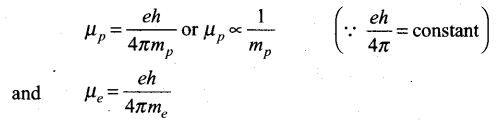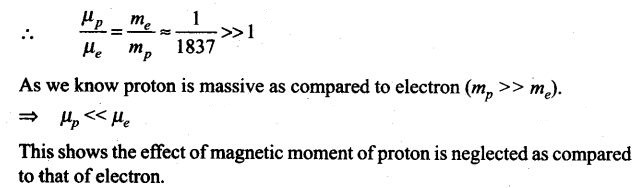Question 12. A permanent magnet in the shape of a thin cylinder of length 10 cm has M = 106 A/m. Calculate the magnetisation current IM.
Solution: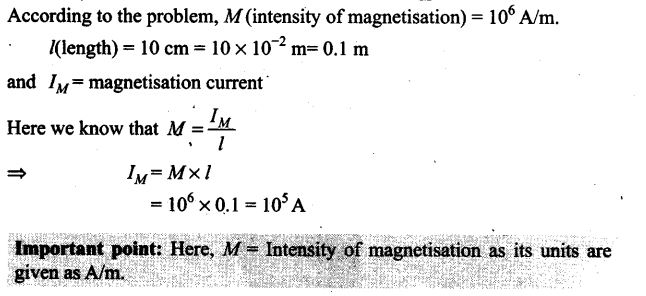Question 13. Explain quantitatively the order of magnitude difference between the diamagnetic susceptibility of N2 (~5 X 10-9) (at STP) and Cu (~10-5).
Solution:
Key concept: Magnetic susceptibility: It is the property of the substance which shows how easily a substance can be magnetised. It can also be defined as the ratio of intensity of magnetisation (I) in a substance to the magnetic intensity (H) applied to the substance, i.e., XM =I/H.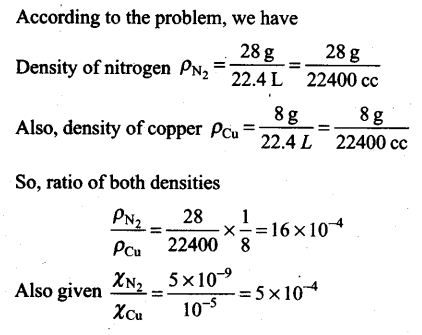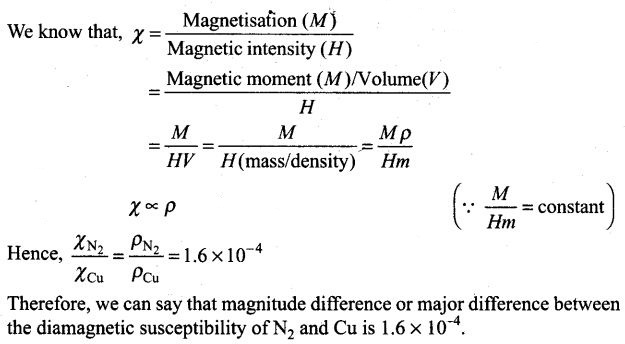Question 14. From molecular view point, discuss the temperature dependence of Susceptibility for diamagnetism, paramagnetism and ferromagnetism.
Solution: Diamagnetism is due to the orbital motion of electrons in an atom developing magnetic moments opposite to applied field. Thus, the resultant magnetic moment of the diamagnetic material is zero, and hence the susceptibility x of diamagnetic material is not much affected by temperature.
Paramagnetism and ferromagnetism is due to alignments of atomic magnetic moments in the direction of the applied field. As temperature is raised, the alignment is disturbed, resulting decrease in susceptibility of both with increase in temperature.

Question 15. A ball of superconducting material is dipped in liquid nitrogen and placed near a bar magnet.
(i) In which direction will it move?
(ii) What will be the direction of its magnetic moment?
Solution:
Key concept: A superconducting material and nitrogen both are diamagnetic in nature.
When a diamagnetic material is dipped in liquid nitrogen, it again behaves as a diamagnetic material. Thus, superconducting material will again behave as a diamagnetic material. When this diamagnetic material is placed near a bar magnet, it will be feebly magnetised opposite to the direction of magnetising field.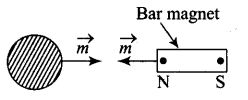(i) So it will move away from the magnet.
(ii) Magnetic moment is from left to right and it is opposite to the direction of magnetic field.

Question 16. Verify the Gauss’s law for magnetic field of a point dipole of dipole moment m at the origin for the surface which is a sphere of radius R.
Solution: Let us draw the figure for given situation,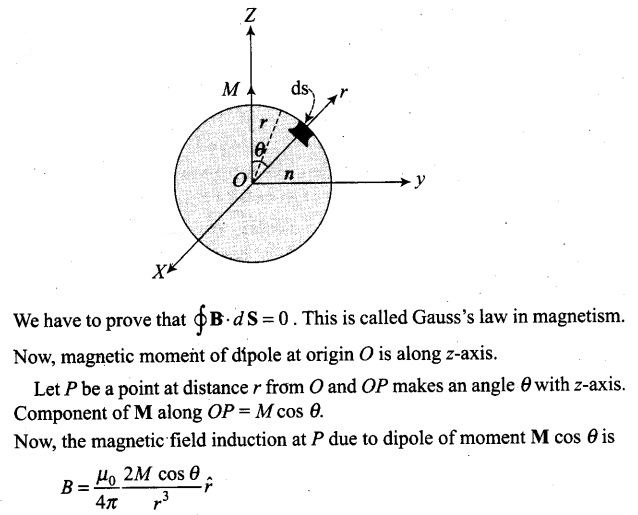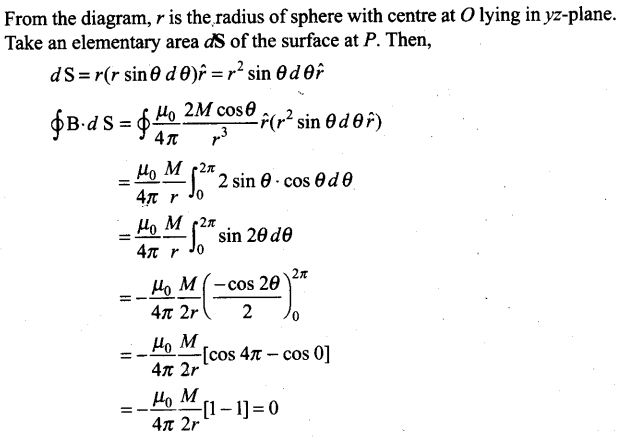Question 17. Three identical bar magnets are rivetted together at centre in the same plane as shown in figure. This system is placed at rest in a slowly varying magnetic field. It is found that the system of magnets does not show any motion. The north-south poles of one magnet is shown in the figure. Determine the poles of the remaining two.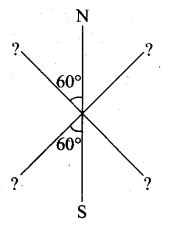Solution: If the net force on the system is zero and net torque on the system is also zero, then the system will be in stable equilibrium. This is possible only when the poles of the remaining two magnets are as shown as below.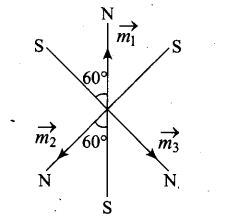Question 18. Suppose we want to verify the analogy between electrostatic and magnetostatic by an explicit experiment. Consider the motion of (i) electric dipole p in an electrostatic field E and (ii) magnetic dipole M in a magnetic field B. Write down a set of conditions on E, B, p, M so that the two motions are verified to. be identical. (Assume identical initial conditions).
Solution: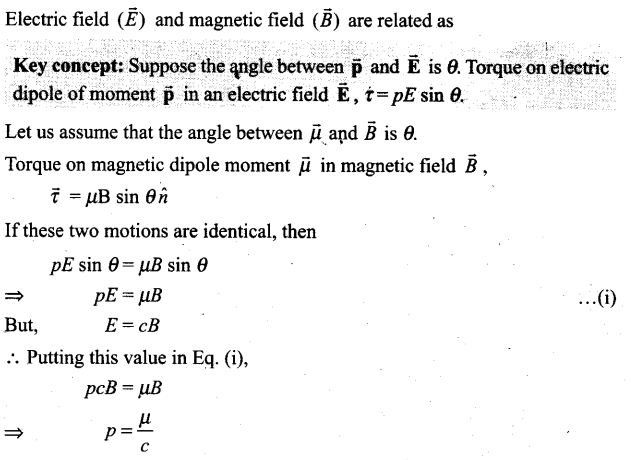Question 19. A bar magnet of magnetic moment M and moment of inertia 1 (about centre, perpendicular to length) is cut into two equal pieces, perpendicular to length. Let T be the period of oscillations of the original magnet about an axis through the mid-point, perpendicular to length, in a magnetic field B. What would be the similar period T for each piece?
Solution: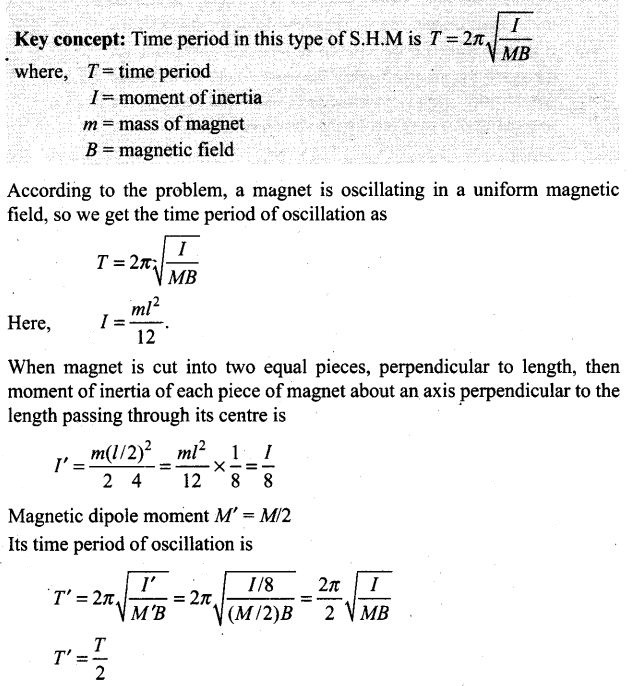Question 20. Use (i) the Ampere’s law for H and (ii) continuity of lines of B, to conclude that inside a bar magnet, (a) lines of H run from the A-pole to S-pole, while (b) lines of B must run from the S-pole to A-pole.
Solution: Let us consider a magnetic field line of B through the bar magnet as given in the figure below. It must be a closed loop.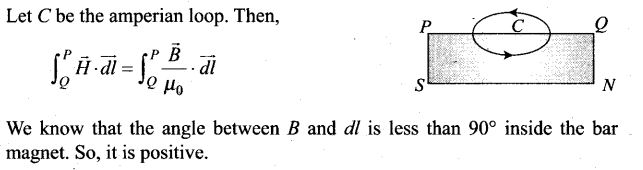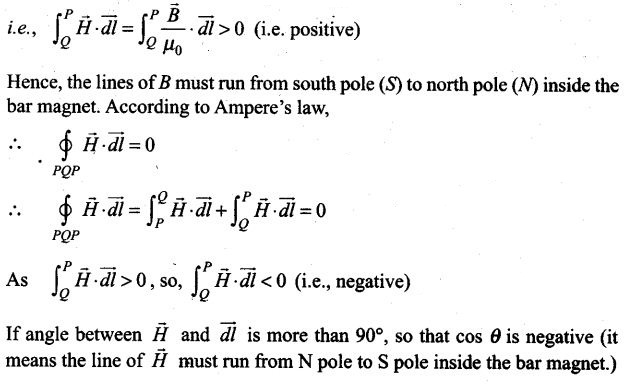Question 21. Verify the Ampere’s law for magnetic field of a point dipole of dipole moment M= Mk. Take C as the closed curve running clockwise along
(i) the z-axis from z = a > 0 to z = R,
(ii) along the quarter circle of radius R and centre at the origin in the first quadrant of xz-plane,
(iii) along the x-axis from x = R to x – a, and
(iv) along the quarter circle of radius a and centre at the origin in the first quadrant of xz-plane
Solution: Consider a plane on x-z plane on which there are two loops (of radius R and a)and a point dipole on origin of dipole moment M(as shown in the figure). From P to Q, every point on the z-axis lies at the axial line of magnetic dipole of moment M.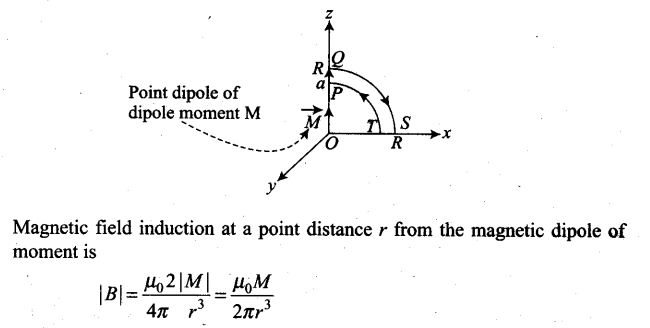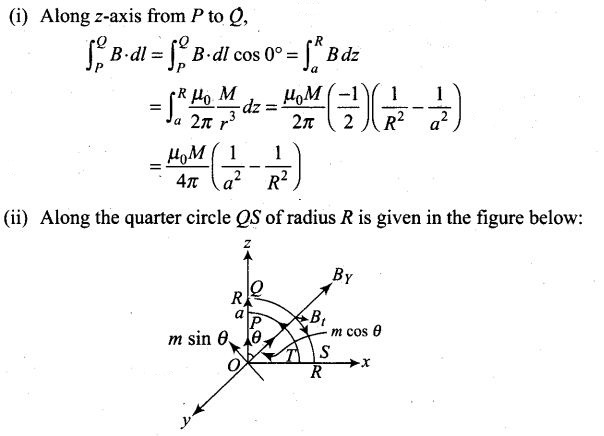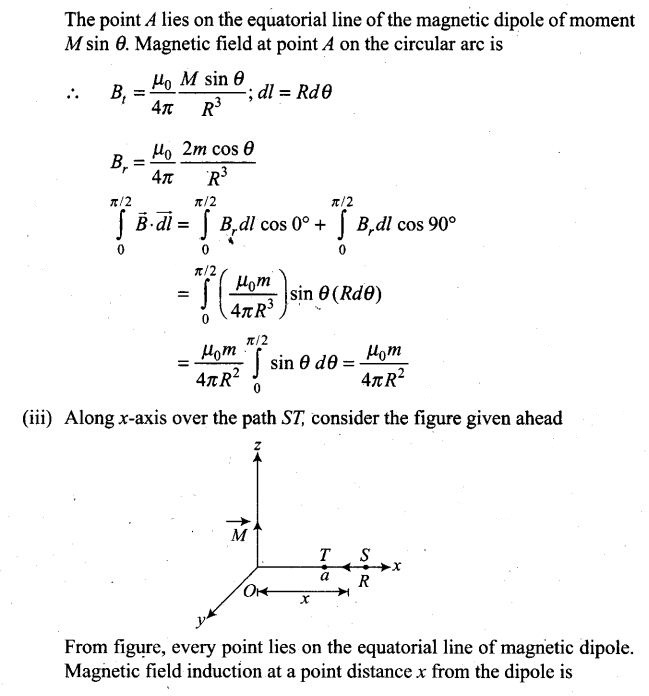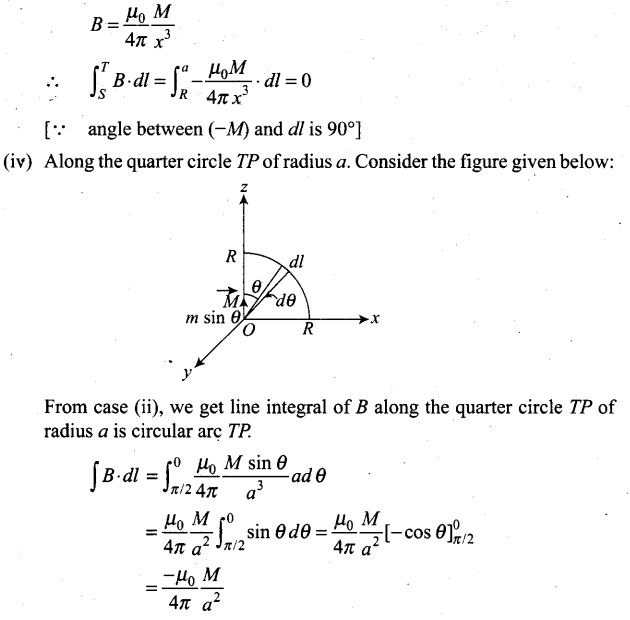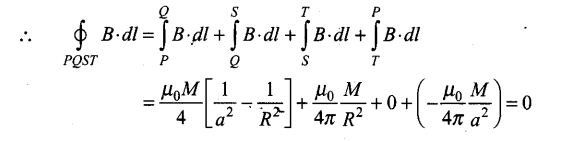Question 22.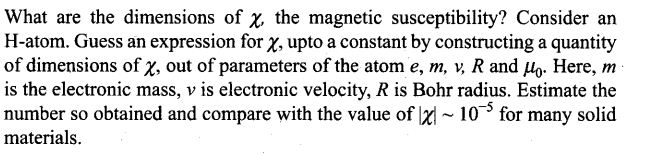Solution: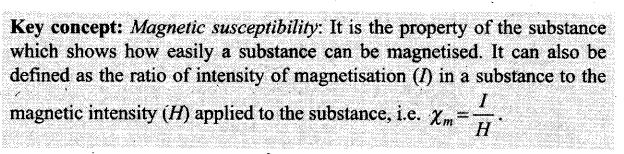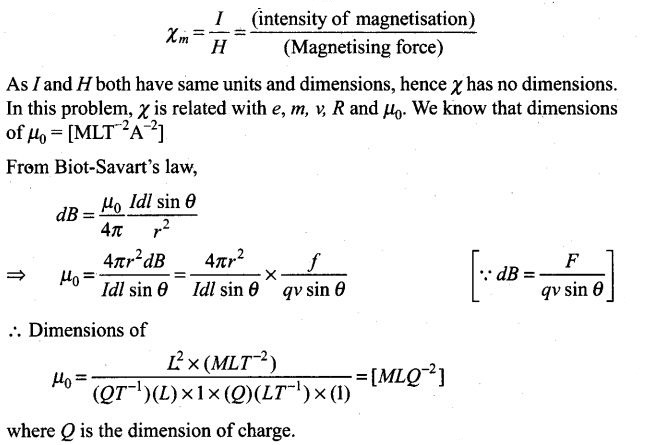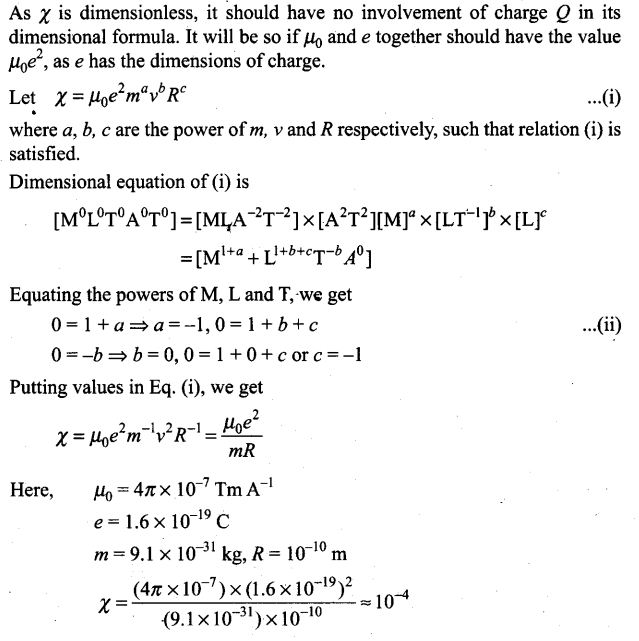Question 23.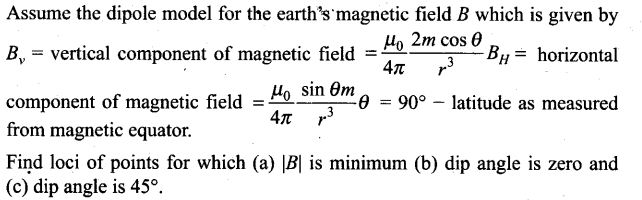Solution: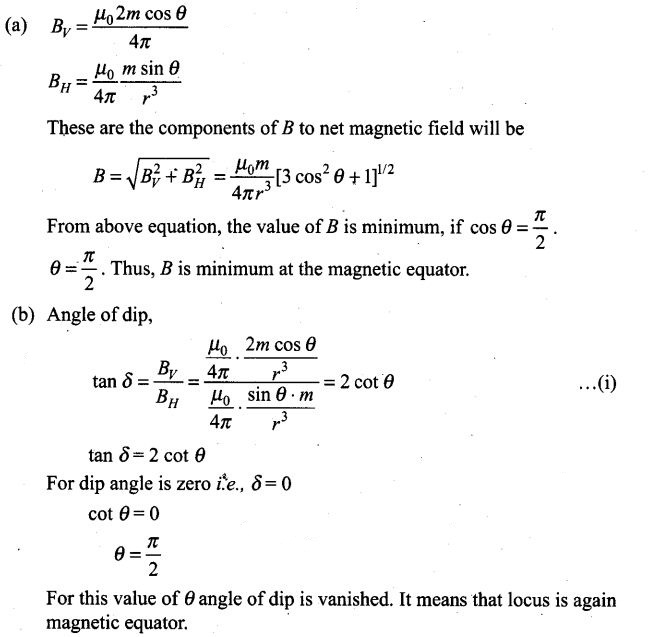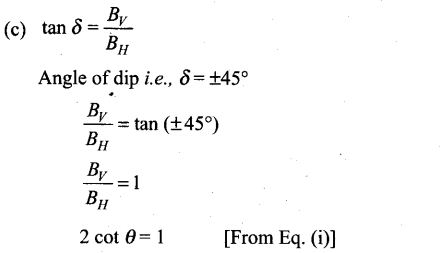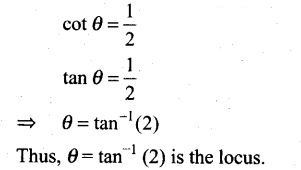Question 24. Consider the plane S formed by the dipole axis and the axis of earth. Let P be a point on the magnetic equator and in S. Let Q be the point of intersection of the geographical and magnetic equators. Obtain the declination and dip angles at P and Q.
Solution: Let point P is in the plane, S needle is in north, so the declination is zero.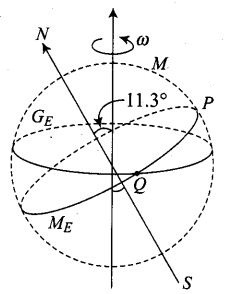From figure,
For point P : Since point P lies in plane S formed by the dipole axis and the axis of the Earth, declination is zero,
For point Q : Since point Q lies on the magnetic equator, angle of dip is zero. Thus the angle of declination is 11.3°

Question 25. There are two current carrying planar coil made each from identical wires of length L. C1 is circular (radius R) and C2 is square (side a). They are so constructed that they have same frequency of oscillation when they are placed in the same uniform B and carry the same current. Find a in terms of R.
Solution: